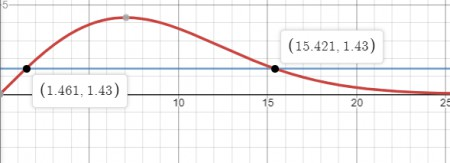# Suppose a study indicates that t years from now, the level of carbon dioxide in the air of a...

## Question:

Suppose a study indicates that t years from now, the level of carbon dioxide in the air of a certain city will be

{eq}L(t) = te^{-.01t^2} {/eq}

parts per million (ppm) for {eq}0 \leq t \leq 20 {/eq}?

(a) Find the average level of carbon dioxide in the first three years _____ ppm

(b) Find the two times {eq}t_1<t_2 {/eq} (in years) that the average level of carbon dioxide actually occurs. (give answers to the nearest one hundredth of a year)

{eq}t_1= {/eq} _____

{eq}t_2= {/eq} _____

## Average Value of a Function:

The average value of a function {eq}f(x) {/eq} over the interval {eq}\displaystyle a {/eq} to {eq}\displaystyle b {/eq} is given by the formula:

$$f_{ave}=\frac{1}{b-a} \int_a^b f(x)dx$$

Apart from this formula, we also use the substitution method of integration to help us along with the integration. All this is shown below.

A

The average value of a function, {eq}\displaystyle f(x) {/eq} over the interval {eq}\displaystyle a {/eq} to {eq}\displaystyle b {/eq} is given by the formula:

$$f_{ave}=\frac{1}{b-a} \int_a^b f(x)dx$$

We apply this formula to the given function.

We can apply a substitution to help us with the integration. We allow {eq}\displaystyle u = -0.01t^2 {/eq} such that {eq}\displaystyle du = -0.02t\,dt {/eq} or {eq}\displaystyle dt=-\frac{du}{0.02t} {/eq}. The interval of the integral also changes accordingly such that {eq}\displaystyle 0\to -0.01(0)^2 = 0 {/eq} and {eq}\displaystyle 3\to -0.01(3^2) =-0.09 {/eq} We perform the substitution and then proceed with the integration.

\begin{align} \displaystyle f_{ave} &= \frac{1}{b-a} \int_a^b L(t)\,dt\\ &= \frac{1}{3}\int_0^3 te^{-0.01t^2}dt\\ &\text{Making the substitution,}\\ &=\frac{1}{3}\int_0^{-0.09} \frac{e^u}{-0.02}\,du\\ &= -\frac{1}{0.06}\left [e^u \right ]_0^{-0.09}\\ &= 1.43 \end{align}

B)

We can find the values of t for which the function takes the value 1.43 by looking at the graph below.As we can see from the graph the function takes the value 1.43 after 1.461 years and 15.421 years.